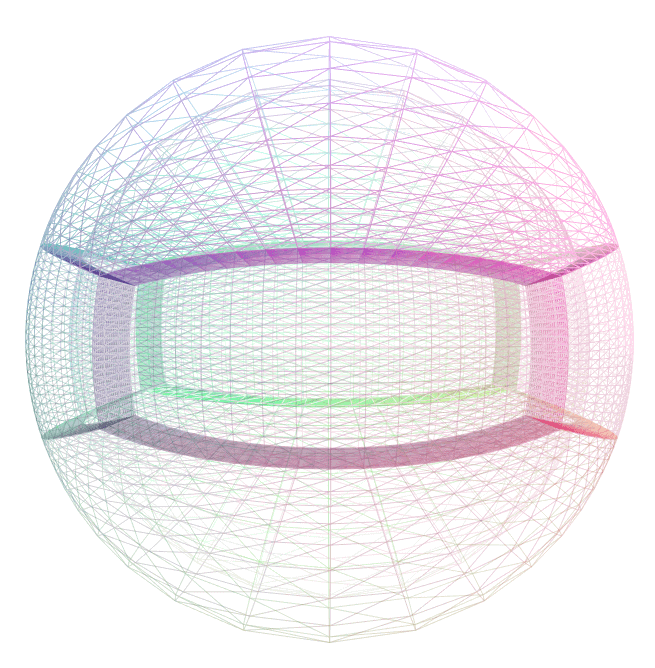SpECTRE  v2023.09.07
domain::CoordinateMaps::EquatorialCompression Class Reference

Redistributes gridpoints on the sphere. More...

#include <EquatorialCompression.hpp>

## Public Member Functions

EquatorialCompression (double aspect_ratio, size_t index_pole_axis=2)

EquatorialCompression (EquatorialCompression &&)=default

EquatorialCompression (const EquatorialCompression &)=default

EquatorialCompressionoperator= (const EquatorialCompression &)=default

EquatorialCompressionoperator= (EquatorialCompression &&)=default

template<typename T >
std::array< tt::remove_cvref_wrap_t< T >, 3 > operator() (const std::array< T, 3 > &source_coords) const

std::optional< std::array< double, 3 > > inverse (const std::array< double, 3 > &target_coords) const
The inverse function is only callable with doubles because the inverse might fail if called for a point out of range, and it is unclear what should happen if the inverse were to succeed for some points in a DataVector but fail for other points.

template<typename T >
tnsr::Ij< tt::remove_cvref_wrap_t< T >, 3, Frame::NoFramejacobian (const std::array< T, 3 > &source_coords) const

template<typename T >
tnsr::Ij< tt::remove_cvref_wrap_t< T >, 3, Frame::NoFrameinv_jacobian (const std::array< T, 3 > &source_coords) const

void pup (PUP::er &p)

bool is_identity () const

## Static Public Attributes

static constexpr size_t dim = 3

## Friends

bool operator== (const EquatorialCompression &lhs, const EquatorialCompression &rhs)

## Detailed Description

Redistributes gridpoints on the sphere.A sphere with an aspect_ratio of 3.

### Details

A mapping from the sphere to itself which redistributes points towards (or away from) a user-specifed axis, indicated by index_pole_axis_. Once the axis is selected, the map is determined by a single parameter, the aspect_ratio $$\alpha$$, which is the ratio of the distance perpendicular to the polar axis to the distance along the polar axis for a given point. This parameter name was chosen because points with $$\tan \theta = 1$$ get mapped to points with $$\tan \theta' = \alpha$$. In general, gridpoints located at an angle $$\theta$$ from the pole are mapped to a new angle $$\theta'$$ satisfying $$\tan \theta' = \alpha \tan \theta$$.

For an aspect_ratio greater than one, the gridpoints are mapped towards the equator, leading to an equatorially compressed grid. For an aspect_ratio less than one, the gridpoints are mapped towards the poles. Note that the aspect ratio must be positive.

Suppose the polar axis were the z-axis, given by index_pole_axis_ == 2. We can then define the auxiliary variables $$r := \sqrt{x^2 + y^2 +z^2}$$ and $$\rho := \sqrt{x^2 + y^2 + \alpha^{-2} z^2}$$.

The map corresponding to this transformation in cartesian coordinates is then given by:

$\vec{x}'(x,y,z) = \frac{r}{\rho}\begin{bmatrix} x\\ y\\ \alpha^{-1} z\\ \end{bmatrix}.$

The mappings for polar axes along the x and y axes are similarly obtained.

The documentation for this class was generated from the following file:
• src/Domain/CoordinateMaps/EquatorialCompression.hpp